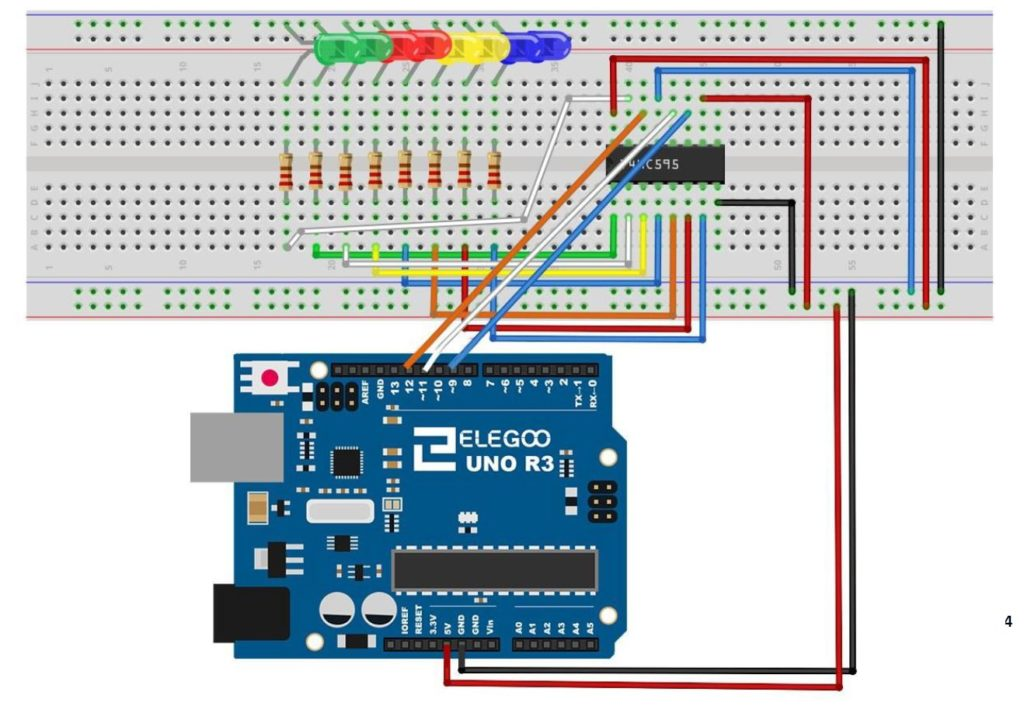Arduino Tutorial 45: Understanding Circular Shift Left and Circular Shift Right with the 74HC595

In this lesson we explore the Circular Shift Left (CSL) and Circular Shift Right (CSR)  binary functions. These functions are similar to the Logical Shift Left and Logical Shift Right functions explained in the previous lesson.

As a reminder, we are doing this work on the circuit built in Tutorial 42. As a refresher, we are using the 74HC595 Serial to Parallel converter, connected to an Arduino according to the following schematic.This is the schematic we use in this example to control 8 LEDs from the 74HC595 chip.

In all these lessons we are using the Arduino Super Starter Kit, which you can pick up HERE.

The Circular Shift Left function is illustrated below:

You can see that all bits shift to the left, with the Most Significant Bit, or MSB looping back to the Least Significant Bit, or LSB position.

Similarly, the Circular Shift Right Function is as follows:

There is actually a very easy way to implement this in a program. We illustrate it with an arduino program. Lets first consider the CSL function. We will first consider how to move the MSB to the LSB position. Remember from our earlier lessons, that dividing a binary number by 2 moves the bits to the right. We want to move the MSB 7 positions to the right, so we would divide by 27, which is 128.  So, if we have a binary number myByte, we could move the MSB to the LSB by the equation:

myByte=myByte/128

With this we do the hardest part, which is to get the MSB to the LSB. Now we need to get the rest of the bits back, and in the left shifted position, we do that by multiplying by two. By combining these two functions, we end up with the solution of CSL being:

myByte=myByte/128+myByte*2

This simple equation will perform the CSL operation, when myByte is a Hexadecimal or Binary number (Byte type in Arduino).

We show the code below to implement the CSL an arduino with a 74HC595 chip:

Similarly, the CSR binary function can be achieved with the equation:

myByte=myByte*128+myByte/2

This is the code we use for CSR on the Arduino.